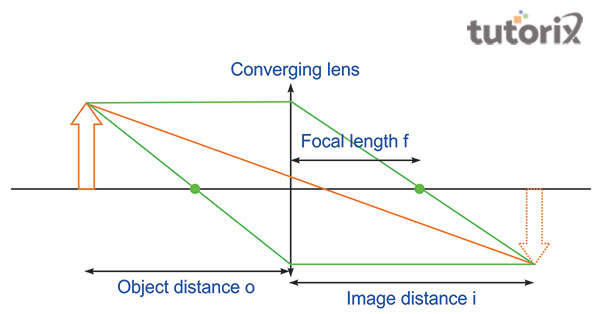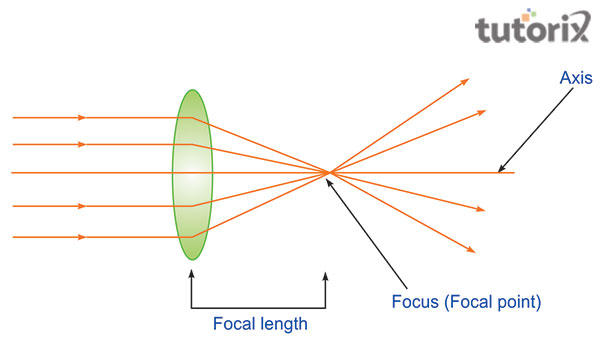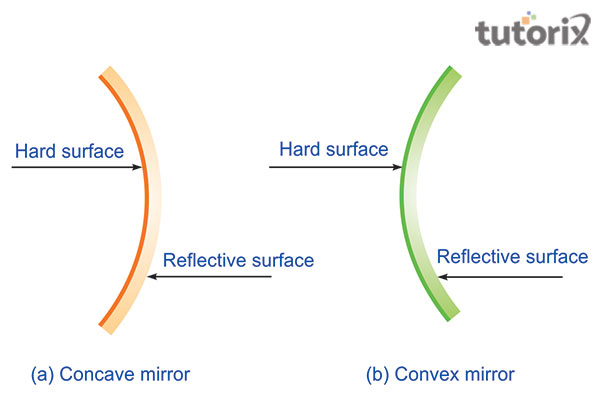# Determination of Focal Length of Concave Mirror and Convex Lens

## Introduction

The distance between an object and the mirror is subject to measure by applying different theories of mirrors and lenses. The law of reflection is one of the essential rules to measure the focal length. In this situation, where the image is formed by the consistency of a surface’s reflection due to the inside curved of a spherical mirror, it will follow the law of reflection of light. In this tutorial, information regarding concave mirrors and the convex lens are subject to discussion.

A reflecting surface of a spherical mirror is curved from the inside and it can obey the reflection law for light like a plane mirror. Mirrors, where the consistency of the reflecting surface makes the plane mirrors to the converging ones, are the concave mirror. The concave mirror can reflect the light inward way to one focal point.

The utilisation of this mirror is primarily for focusing light on a specific point. In this mirror, the type of image depends on the length between the mirror and the placed object at the same line.

The rays of light that are coming from a specific distance are parallel to meet the specific points on the mirror’s front. Additionally, the prior use of the concave mirror is, that this can assist in the identification purpose of the position of the actual image depending on the position of the lens.Figure 1: Principal of Concave mirror

## Description of Convex Lens

The lens that helps in arranging the position of the lens where the distance with the image is helping in determining the value of the focal length is the convex lens. When the curve of the mirror is from the outside in a spherical surface, the convex lens occurs in that place, which is valuable to understanding the object’s distance from the lens.

These lenses can reduce the light arriving from the outside and it enables users to focus on a point to receive the obvious vision. The point is the focus point (f) which helps in acknowledging the distance of an image from the spherical mirror.Figure 2: Convex Lens

## Focal Length of a Concave Mirror

A concave mirror refers to the spherical mirror which can reflect the curved surface inside and follow the reflection of the law of light. The coming light from an object that is placed at a distance is taken into consideration to be parallel. The above-furnished process is adequate to find the focal length using a concave mirror.

## Theory

A spherical mirror can reflect when the surface where the curve is in place the inward to forward the focus. According to physics, the concave mirrors are identical as they do follow the reflection of light law all the time. In the situation, when the light comes from the incident ray as a parallel to the surface, the converging ray at a point is known as the principal focus of the concave mirror.

The light rays arriving from a distant object are assumed to be parallel. If the formed image is real, inverted and tiny in measurement, then the parallel rays of light encounter the point in the mirror’s front. ‘f’ represents the contrast between the principal axis or ‘P’ and the focus or ‘F’ of the concave mirror. Additionally, images can be acquired on the screen as the formed image is using the real lens (Yasin & Khasanah, 2018). Before experimenting with the process of measuring the focal length, the difference between the concave and convex is essential to comprehend.Figure 3: Concave and Convex mirror

## Materials used

Students who want to experiment with the focus point determination of the focal length are directed to use the following materials:

• A concave mirror

• A holder to fix the mirror position

• A stand for the mirror

• A scale to measure the distance

• A screen holder

With the utilisation of the above-mentioned materials, one can experiment the focal length of the concave mirror.

## Procedure

The distance between the object needs to be placed at a distance of more than 50 feet. The placement of the concave mirror and the mirror stand need to be stood at a specific distance and placed as facing each other. The placement of the screen is one of the vital points which is regarded as the reflecting surface of the mirror. The determination of the experiment is also depending on the metre scale which needs to be placed at a distance between the screen and the mirror. The distance of the mirror needs to be the same as the focal length (Yin et al. 2020). Students need to repeat the same process to estimate the average of the focal length.

## Conclusion

In this tutorial, the determination of the focal length of a concave mirror is highlighted with the specific requirements. The obtained image from the mirror and lens is helpful to construct the experiment for someone. Moreover, the additional knowledge regarding the concave mirror use cases and the procedures that are followed in the reflection of light laws is essential to construct the topic. Different precautions are required to have such as the placement of the concave mirror need to face the window, and the measuring scale needs to be perpendicular in the position with the holder of the mirror to avoid errors.

## FAQs

Q1. What is Focal Length of a Convex Mirror?

Ans. The primary characteristic of the convex mirror is that it is thicker in the middle portion and thinner on the edge. Convex are the converging lens.

Q2. When the light rays are parallel?

Ans. The parallel light rays can meet the point on the mirror’s front, only when the inverted size of the image is small and the image is real.